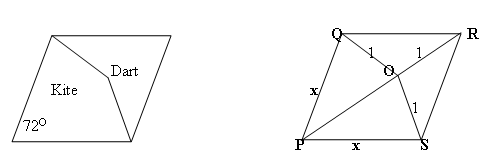#### You may also like### Golden Thoughts

Rectangle PQRS has X and Y on the edges. Triangles PQY, YRX and XSP have equal areas. Prove X and Y divide the sides of PQRS in the golden ratio.### Pent

The diagram shows a regular pentagon with sides of unit length. Find all the angles in the diagram. Prove that the quadrilateral shown in red is a rhombus.### Pentakite

Given a regular pentagon, can you find the distance between two non-adjacent vertices?

# Darts and Kites

##### Age 14 to 16Challenge LevelThis solution is from Arun Iyer of SIA High School and Junior College.

Part 1
First I will show that $POR$ is a straight line. For this I would like to state the perpendicular bisector theorem.

PERPENDICULAR BISECTOR THEOREM: Every point equidistant from the two ends of a line segment lies on the perpendicular bisector of the line segment.

Now consider the line segment $QS$.

$OQ=OS=1$ therefore by the perpendicular bisector theorem, $O$ must lie on the perpendicular bisector of $QS$.

$PQ=PS$ (as the sides of the rhombus are equal), therefore by the perpendicular bisector theorem, $P$ must lie on the perpendicular bisector of $QS$.

$RQ=RS$ (as the sides of the rhombus are equal), therefore by the perpendicular bisector theorem, $R$ must lie on the perpendicular bisector of $QS$.

Now the perpendicular bisector of a line segment is unique and hence $P$, $O$, $R$ must lie on the same perpendicular bisector and hence $POR$ is a straight line.

Part 2
Now I will get all the angles of the rhombus.

$\angle QPS$ = $72^{\circ}$ (given), $\angle QRS = \angle QPS = 72^{\circ}$ as they are opposite angles of a rhombus. The diagonal of the rhombus bisects the angles of a rhombus and therefore $\angle QPO = \angle SPO = \angle QRO = \angle SRO = 36^{\circ}$.

Triangles $OQR$ and $OSR$ are isosceles triangles, therefore $\angle OSR = \angle OQR = 36^{\circ}$.

Using the fact that sum of angles of a triangle is 180 degrees, we can see that $\angle SOR$ and $\angle QOR$ are equal to 108 degrees. Since we have proved that $POR$ is a straight line in Part 1, we can determine $\angle QOP$ and $\angle SOP$ to be 72 degrees.

Again using the fact that sum of angles of a triangle is 180 degrees, we can see that $\angle OQP$ and $\angle OSP$ are equal to 72 degrees.

Part 3

Let the side of the rhombus be $x$.
Consider triangle $OPS$ in which $PO=PS=x$ (since $\angle POS = \angle PSO$).
Applying the cosine rule $$\cos OPS = [PS^2 + PO^2 - OS^2]/[2\times PS \times PO]$$ therefore $$\cos 36 =[2x^2-1]/[2x^2]\quad (1).$$
Splitting the isosceles triangle $ORS$ into two right angled triangles gives $$\cos 36 = x/2 \quad (2).$$
From (1) and (2),
\eqalign{ [2x^2 - 1]/[2x^2] &= x/2\cr x^3 - 2x^2 + 1 &= 0 \cr (x - 1)(x^2 - x - 1) &= 0.}
Now $x\neq 1$ because triangle POS is not equilateral, therefore $x^2 - x - 1 = 0$ and hence
$x = [1 + \sqrt 5]/2$ or $x = [1 - \sqrt 5]/2$.
Clearly $x\neq [1 - \sqrt 5]/2$ because the side length cannot be negative, therefore $x = [1 + \sqrt 5]/2$, the Golden Ratio.